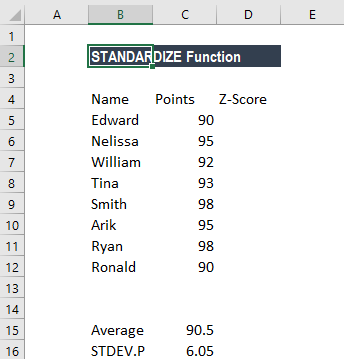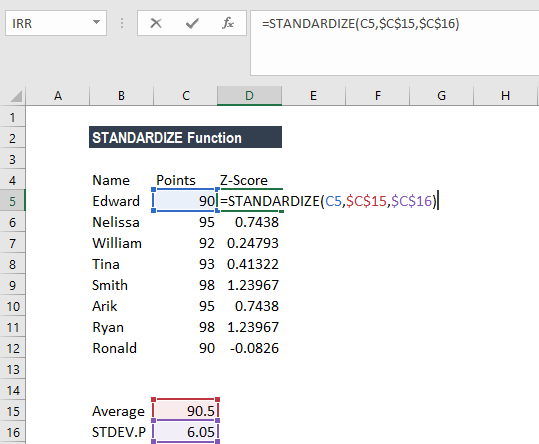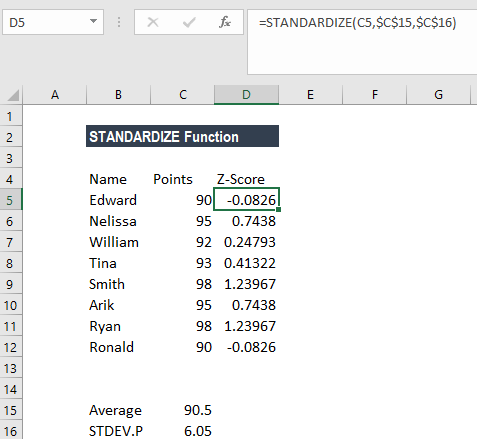# STANDARDIZE Function

Return a normalized value (z-score) based on the mean and standard deviation

## What is the STANDARDIZE Z-Score Function?

The STANDARDIZE Function is available under Excel Statistical functions. It will return a normalized value (z-score) based on the mean and standard deviation. A z-score, or standard score, is used for standardizing scores on the same scale by dividing a score’s deviation by the standard deviation in a data set. The result is a standard score. It measures the number of standard deviations that a given data point is from the mean.

A z-score can be negative or positive. A negative score indicates a value less than the mean, and a positive score indicates a value greater than the mean. The average of every z-score for a data set is zero.

Z-scores are a way to compare results from a test to a “normal” population. The results from tests or surveys can include thousands of possible results and units. However, the results often seem meaningless. For example, knowing that someone’s height is 180 cms. can be useful information. However, if we want to compare it to the “average” person’s height, looking at a vast table of data can be overwhelming (especially if some heights are recorded in feet). A z-score can tell us where that person’s height is, as compared to the population’s mean (average) height.

### Z-Score Formula

=STANDARDIZE(x, mean, standard_dev)

The STANDARDIZE function uses the following arguments:

1. X (required argument) – This is the value that we want to normalize.
2. Mean (required argument) – The arithmetic mean of the distribution.
3. Standard_dev (required argument) – This is the standard deviation of the distribution.

### How to use the STANDARDIZE Z-Score Function in Excel?

To understand the uses of the STANDARDIZE function, let‘s consider an example:

#### Example 1

Suppose we are given the following data:The formula we use is:We get the result below:### A few notes about the STANDARDIZE Function

1. #NUM! error – Occurs if the given standard_dev argument is less than 0.
2. #VALUE! error – Occurs if any of the given arguments are non-numeric.

Thanks for reading CFI’s guide to important Excel functions! By taking the time to learn and master these functions, you’ll significantly speed up your financial analysis. To learn more, check out these additional CFI resources:

• Excel Functions for Finance